Next: Basins of attraction Up: The chaotic pendulum Previous: The Poincaré section

## Spatial symmetry breaking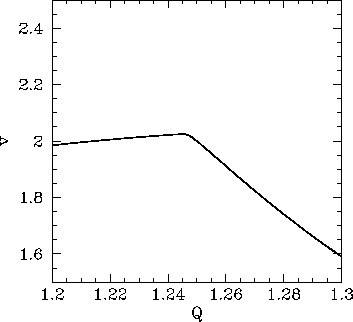Suppose that we now gradually increase the quality-factor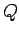. What happens to the simple orbit shown in Fig. 28? It turns out that, at first, nothing particularly exciting happens. The size of the orbit gradually increases, indicating a corresponding increase in the amplitude of the pendulum's motion, but the general nature of the motion remains unchanged. However, something interesting does occur whenis increased beyond about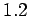. Figure 30 shows the-coordinate of the orbit's Poincaré section plotted againstin the rangeand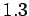. Note the sharp downturn in the curve at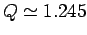. What does this signify? Well, Fig. 31 shows the time-asymptotic phase-space orbit just before the downturn (i.e., at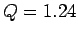), and Fig. 32 shows the orbit somewhat after the downturn (i.e., at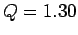). It is clear that the downturn is associated with a sudden change in the nature of the pendulum's time-asymptotic phase-space orbit. Prior to the downturn, the orbit spends as much time in the region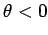as in the region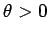. However, after the downturn the orbit spends the majority of its time in the region. In other words, after the downturn the pendulum bob favours the region to the left of the pendulum's vertical. This is somewhat surprising, since there is nothing in the pendulum's equations of motion which differentiates between the regions to the left and to the right of the vertical. We refer to a solution of this type--which fails to realize the full symmetry of the dynamical system in question--as a symmetry breaking solution. In this case, because the particular symmetry which is broken is a spatial symmetry, we refer to the process by which the symmetry breaking solution suddenly appears, as the control parameteris adjusted, as spatial symmetry breaking. Needless to say, spatial symmetry breaking is an intrinsically non-linear process--it cannot take place in dynamical systems possessing linear equations of motion.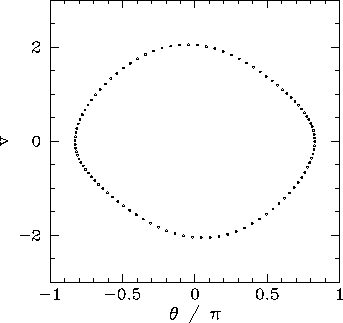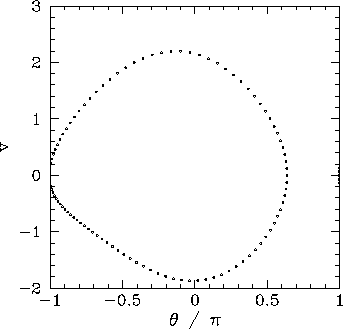It stands to reason that since the pendulum's equations of motion favour neither the left nor the right then the left-favouring orbit pictured in Fig. 32 must be accompanied by a mirror image right-favouring orbit. How do we obtain this mirror image orbit? It turns out that all we have to do is keep the physics parameters,, andfixed, but change the initial conditions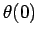and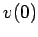. Figure 33 shows a time-asymptotic phase-space orbit calculated with the same physics parameters used in Fig. 32, but with the initial conditions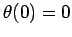and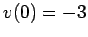, instead ofand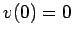. It can be seen that the orbit is indeed the mirror image of that pictured in Fig. 32.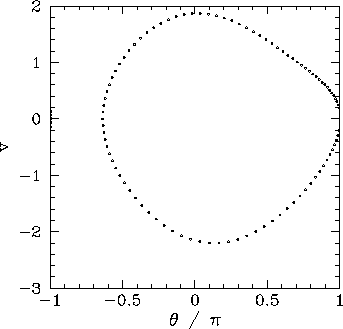Figure 34 shows the-coordinate of the Poincaré section of a time-asymptotic orbit, calculated with the same physics parameters used in Fig. 30, versusin the rangeand. Data is shown for the two sets of initial conditions discussed above. The figure is interpreted as follows. Whenis less than a critical value, which is about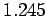, then the two sets of initial conditions lead to motions which converge on the same, left-right symmetric, period-1 attractor. However, onceexceeds the critical value then the attractor bifurcates into two asymmetric, mirror image, period-1 attractors. Obviously, the bifurcation is indicated by the forking of the curve shown in Fig. 34. The lower and upper branches correspond to the left- and right-favouring attractors, respectively.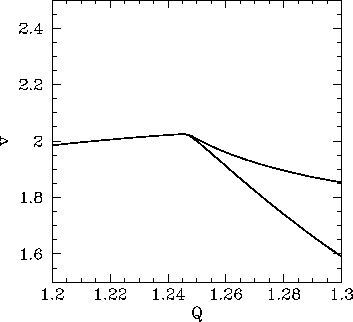Spontaneous symmetry breaking, which is the fundamental non-linear process illustrated in the above discussion, plays an important role in many areas of physics. For instance, symmetry breaking gives mass to elementary particles in the unified theory of electromagnetic and weak interactions.25Symmetry breaking also plays a pivotal role in the so-called inflation'' theory of the expansion of the early universe.26Next: Basins of attraction Up: The chaotic pendulum Previous: The Poincaré section
Richard Fitzpatrick 2006-03-29